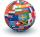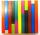# The farmer

The farmer had 140 sheep. For the next year, she decided to change the number of sheep in ratio 10: 7. How many sheep will he have then?

Result

n =  98

#### Solution:

140 = 10/7 * n

10n = 980

n = 98

Calculated by our simple equation calculator.

Leave us a comment of example and its solution (i.e. if it is still somewhat unclear...):

Showing 0 comments:Be the first to comment!#### To solve this verbal math problem are needed these knowledge from mathematics:

Need help calculate sum, simplify or multiply fractions? Try our fraction calculator. Do you have a linear equation or system of equations and looking for its solution? Or do you have quadratic equation?

## Next similar examples:

1. Cleaning windowsCleaning company has to wash all the windows of the school. The first day washes one-sixth of the windows of the school, the next day three more windows than the first day and the remaining 18 windows washes on the third day. Calculate how many windows ha
2. EquationSolve the equation: 1/2-2/8 = 1/10; Write the result as a decimal number.
3. UN 1If we add to an unknown number his quarter, we get 210. Identify unknown number.
4. FractionsThree-quarters of an unknown number are 4/5. What is 5/6 of this unknown number?
5. Unknown numberI think the number - its sixth is 3 smaller than its third.
6. Unknown numberIdentify unknown number which 1/5 is 40 greater than one tenth of that number.
7. TranslationsIf I going to translate the book 6 pages per day I translate it 4 days earlier than if I translated 5 pages a day. If I translate 4 pages a day I translate it for how many days.....?
8. 1.5 divided1.5 divided by 1 = w divided by 4
9. PearsThere were pears in the basket, I took two-fifths of them, and left six in the basket. How many pears did I take?
10. Equation with fractionsSolve equation: ? It is equation with fractions.
11. Equation with xSolve the following equation: 2x- (8x + 1) - (x + 2) / 5 = 9
12. EqnSolve equation with fractions: 2x/3-50=40+x/4
13. Simple equation 6Solve equation with one variable: X/2+X/3+X/4=X+4
14. Simple equationSolve for x: 3(x + 2) = x - 18
15. The rodThe rod is painted in four colors. 55% of the bar is painted in blue, green 0.2 of rod, 1/8 is brown and the remaining 45 cm of white. How long is rod?
16. PlayingHow long have we trained on the pitch when we know that the warm-up took 10 minutes, we trained passes for one-third of the time and we played football half the time?
17. SeamstressThe seamstress cut the fabric into 3 parts. The first part was the eighth fabric, the second part was three-fifths of the fabric and the third part had a length of 66 cm. Calculate the original length of the fabric.